Ex 5.3

Chapter 5 Class 10 Arithmetic Progressions
Serial order wise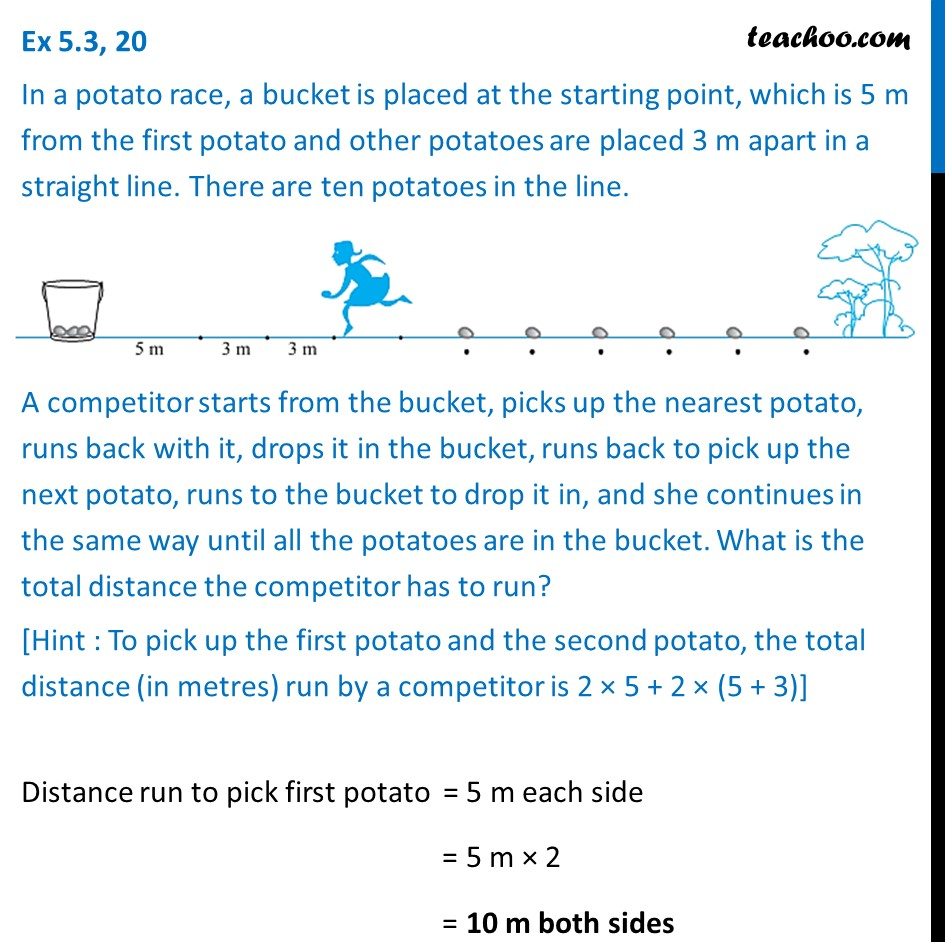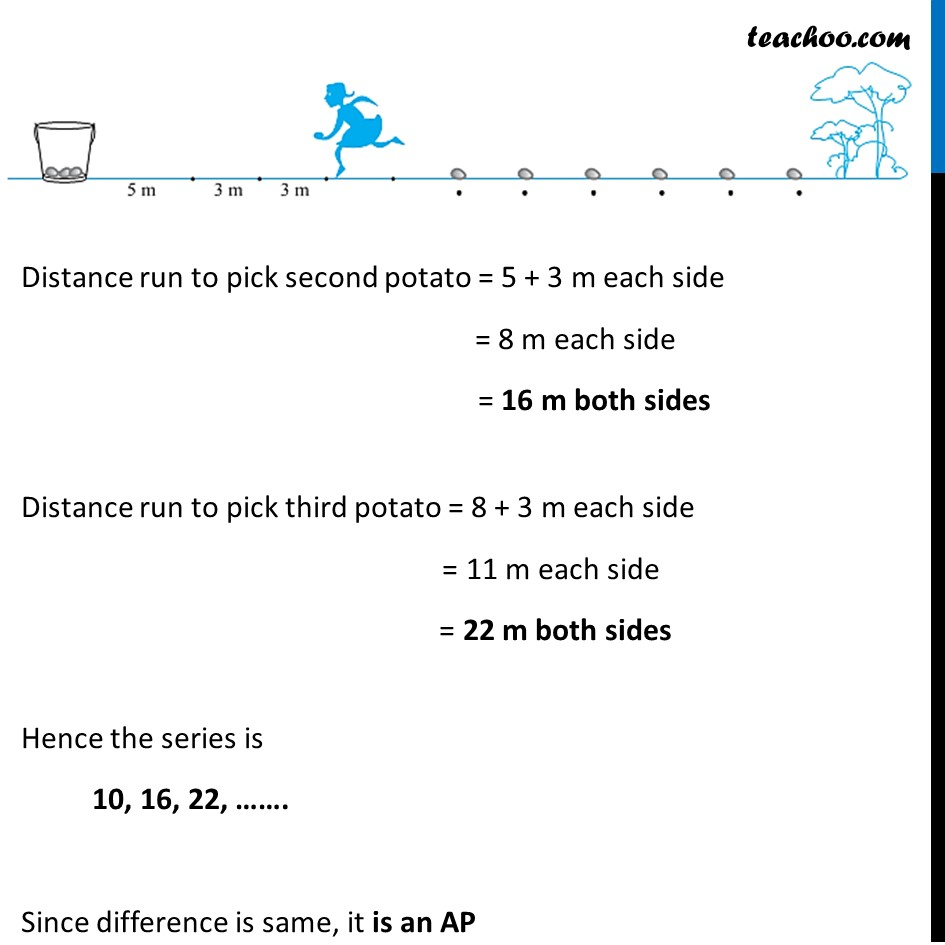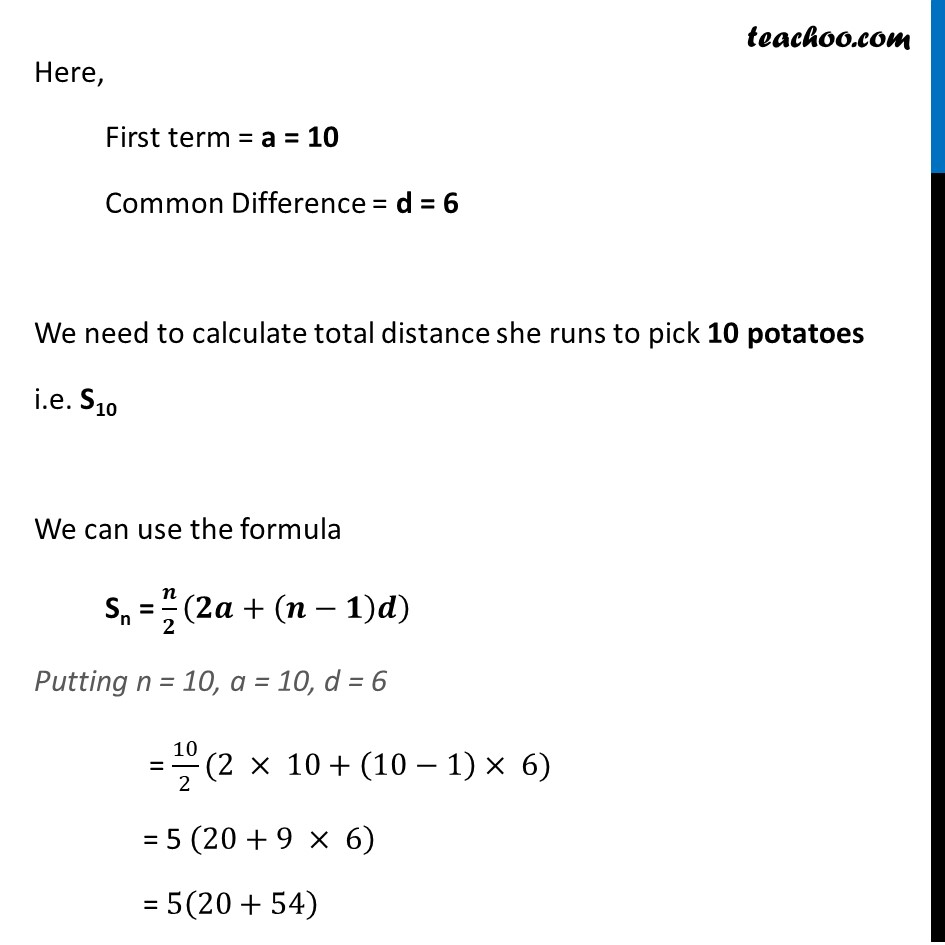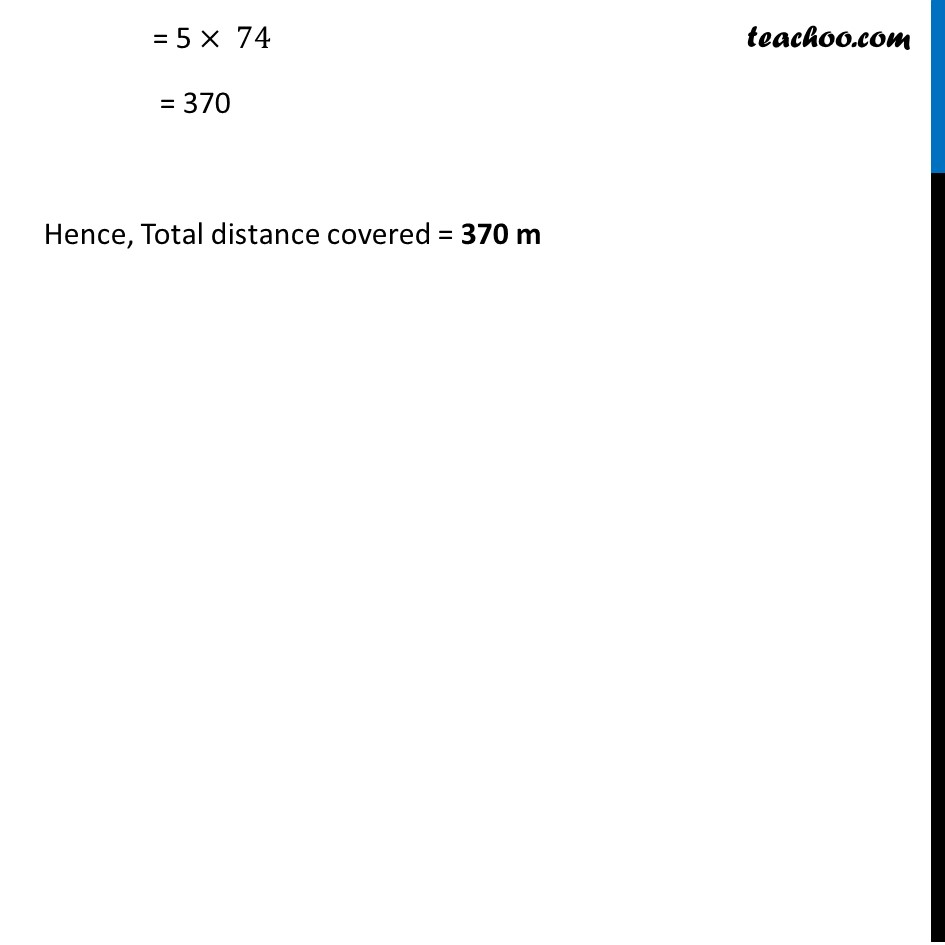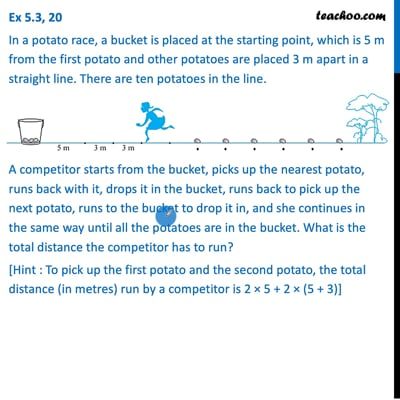This video is only available for Teachoo black users

Solve all your doubts with Teachoo Black (new monthly pack available now!)

### Transcript

Ex 5.3, 20 In a potato race, a bucket is placed at the starting point, which is 5 m from the first potato and other potatoes are placed 3 m apart in a straight line. There are ten potatoes in the line. A competitor starts from the bucket, picks up the nearest potato, runs back with it, drops it in the bucket, runs back to pick up the next potato, runs to the bucket to drop it in, and she continues in the same way until all the potatoes are in the bucket. What is the total distance the competitor has to run? [Hint : To pick up the first potato and the second potato, the total distance (in metres) run by a competitor is 2 × 5 + 2 × (5 + 3)] Distance run to pick second potato = 5 + 3 m each side = 8 m each side = 16 m both sides Distance run to pick third potato = 8 + 3 m each side = 11 m each side = 22 m both sides Hence the series is 10, 16, 22, ……. Since difference is same, it is an AP Here, First term = a = 10 Common Difference = d = 6 We need to calculate total distance she runs to pick 10 potatoes i.e. S10 We can use the formula Sn = 𝒏/𝟐 (𝟐𝒂+(𝒏−𝟏)𝒅) Putting n = 10, a = 10, d = 6 = 10/2(2 × 10+(10−1)× 6) = 5 (20+9 × 6) = 5(20+54) = 5 × 74 = 370 Hence, Total distance covered = 370 m